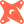Introducing PopSQL + dbt. The first SQL editor with built-in dbt support.Read more →

# How to Get the First Row per Groupin PostgreSQL

Let's say we have an `events` table that belongs to a `user_id`, and we want to see the first event for each user for that day. The function we need here is `row_number`. It's got a tricky syntax that I always forget. Here's an example PostgreSQL query:

``````select
*,
row_number() over (partition by user_id order by created_at desc) as row_number
from events
where day = '2018-01-01'::date``````

This gives us all the event IDs for the day, plus their `row_number`. Since we only want the first event for the day, we only want rows that have `row_number: 1`. To do that, we can use a common table expression:

``````with _events as (
select
*,
row_number() over (partition by user_id order by created_at desc) as row_number
from events
where day = '2018-01-01'::date
)

select *
from _events
where row_number = 1``````

# Ready for a modern SQL editor?